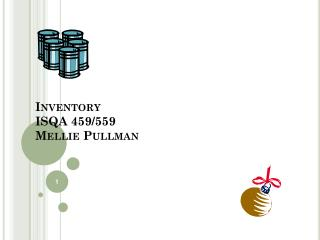DownloadDownload PresentationInventory ISQA 459/559 Mellie Pullman

# Inventory ISQA 459/559 Mellie Pullman

Download Presentation## Inventory ISQA 459/559 Mellie Pullman

- - - - - - - - - - - - - - - - - - - - - - - - - - - E N D - - - - - - - - - - - - - - - - - - - - - - - - - - -
##### Presentation Transcript

1. Inventory ISQA 459/559Mellie Pullman

2. Inventory Costs • Holding (or carrying) costs • Setup (or production change) costs • Ordering costs. • Shortage costs.

3. Inventory costs • C = Unit cost or production cost: the additional cost for each unit purchased or produced. • H = Holding costs: cost of keeping items in inventory(cost of lost capital, taxes and insurance for storage, breakage, etc., handling and storing) • S = Setup or ordering costs: a fixed cost incurred every time you place an order or a batch is produced.

4. Inventory Game Round ONE

5. Total costs of carrying inventory • Assumptions • demand is constant and uniform throughout the period for your products (5 cases per day) • Price per unit is constant for the period (\$16/case) • Inventory holding cost is based on an average cost. • Total Inventory Policy Cost annually= annual purchase cost + annual order cost + annual holding cost

6. Total cost of Inventory Policy • = annual purchase cost (annual demand * Cost/item)+ annual order cost (annual # orders * Cost to order)+ annual holding cost (average units held*cost to carry one unit)

7. Total Inventory Cost Equation D = yearly demand of units C = cost of each unit Q = quantity ordered S = cost to place order H = average yearly holding cost for each unit = storage+interest*C D/Q = number of orders per year Q/2 = average inventory held during a given period assuming with start with Q and drop to zero before next order arrives (cycle inventory).

8. Deriving the EOQ :Economic Order Quantity • Setting the total holding cost equal to the total setup cost and determining Q:

9. Number of units on hand Q Q Q R L L Time R = Reorder point Q = Economic order quantity L = Lead time EOQ Model--Basic Fixed-Order Quantity Model (Q)

10. The Reorder Point Reorder point = (average period demand)*Lead Time periods = d * L

11. Another EOQ Example Annual Demand = 1,000 units Days per year considered in average daily demand = 365 Cost to place an order = \$10 Holding cost per unit per year = \$2.50 Lead time = 7 days Cost per unit = \$15 Determine the economic order quantity & reorder point.

12. Minor Deviations Here • What causes minor deviations from the ideal order size? • Assumptions behind the regular EOQ Model?

13. Inventory Game Round Two:Variable demand

14. Variations in lead time • If we have variations in lead time, how should we change the reorder point so we rarely run out? Reorder Point = Average demand during lead time(d*L) + safety stock (Z* sL) • where: d = average daily (or weekly) demandL = Lead time (matching days or weeks)sL = standard deviation of demand during lead time. sD = standard deviation of demand (days or weeks).

15. Example • Annual Demand = 1000 units • 250 work days in the yeard=1000/250 = 4 units/day • Q= 200 unitsL=9 days sL = 3 units • z=2 (97.7% likelihood that we won’t run out during lead time)Reorder point= d*L +z*sL= (4*9) + (2*3) = 42 units

16. P Method (periodic review) • You have a predetermined time (P) between orders • (sales rep comes by every 10 days) • or the average time between orders from EOQ is Q/D (Q=100 orders; D =1200 orders per year so P=Q/D = 1/12 year or every month. • How much should you order to bring inventory level up to some predetermined level, R?

17. P Method (periodic review) • R = restocking level • Current Inventory position = IP • Order Quantity= R-IP • How do we determine R?

18. Restocking Level • Needs to meet most demand situations • R= Restocking level = Average demand during lead time & review period+ safety stock= mP+L + z* sP+Lwhere:mP+L = average demand during lead time and review period z = # of standard dev from mean above the average demand (higher z is lower probability of running out). sRP+L= standard deviation of demand during lead time + review period